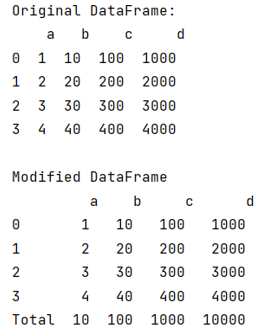# Appending Column Totals to a Pandas DataFrame

Given a DataFrame with numerical values, we need to append a row that represents the sum of each column.
Submitted by Pranit Sharma, on July 21, 2022

Pandas is a special tool that allows us to perform complex manipulations of data effectively and efficiently. Inside pandas, we mostly deal with a dataset in the form of DataFrame. DataFrames are 2-dimensional data structures in pandas. DataFrames consist of rows, columns, and data.

To append a row consisting of the total of column values, we will first create a new column and assign its values as the use of the sum() method.

To work with pandas, we need to import pandas package first, below is the syntax:

```import pandas as pd
```

Let us understand with the help of an example,

## Python code to append column totals to a pandas dataframe

```# Importing pandas package
import pandas as pd

# Creating a Dictionary
d = {
'a':[1,2,3,4],
'b': [10,20,30,40],
'c':[100,200,300,400],
'd': [1000,2000,3000,4000]
}

# Creating a DataFrame
df = pd.DataFrame(d)

# Display original DataFrame
print("Original DataFrame:\n",df,"\n")

# Creating new column and assigning sum of column values
df.loc['Total']= df.sum(numeric_only=True, axis=0)

# Display result
print("Modified DataFrame\n",df)
```

Output:What's New (MCQs)

Top Interview Coding Problems/Challenges!

IncludeHelp's Blogs

Languages: » C » C++ » C++ STL » Java » Data Structure » C#.Net » Android » Kotlin » SQL
Web Technologies: » PHP » Python » JavaScript » CSS » Ajax » Node.js » Web programming/HTML
Solved programs: » C » C++ » DS » Java » C#
Aptitude que. & ans.: » C » C++ » Java » DBMS
Interview que. & ans.: » C » Embedded C » Java » SEO » HR
CS Subjects: » CS Basics » O.S. » Networks » DBMS » Embedded Systems » Cloud Computing
» Machine learning » CS Organizations » Linux » DOS
More: » Articles » Puzzles » News/Updates